## Series Solutions: First ExamplesLet us look (again) at the example

y''+4y=0.

Using other techniques it is not hard to see that the solutions are of the form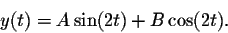We want to illustrate how to find power series solutions for a second-order linear differential equation.

The generic form of a power series is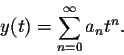We have to determine the right choice for the coefficients (an).

As in other techniques for solving differential equations, once we have a "guess" for the solutions, we plug it into the differential equation. Recall from the previous section that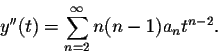Plugging this information into the differential equation we obtain: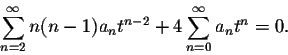Our next goal is to simplify this expression such that only one summation sign "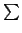" remains. The obstacle we encounter is that the powers of both sums are different, tn-2 for the first sum and tn for the second sum. We make them the same by shifting the index of the first sum by 2 units to obtain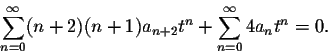Now we can combine the two sums as follows: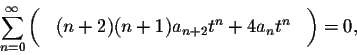and factor out tn: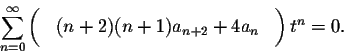Next we need a result you probably already know in the case of polynomials: A polynomial is identically equal to zero if and only if all of its coefficients are equal to zero. This results also holds true for power series:

Theorem. A power series is identically equal to zero if and only if all of its coefficients are equal to zero.

This theorem applies directly to our example: The power series on the left is identically equal to zero, consequently all of its coefficients are equal to 0: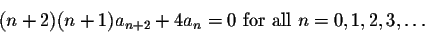Solving these equations for the "highest index" n+2, we can rewrite as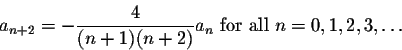These equations are known as the "recurrence relations" of the differential equations. The recurrence relations contain all the information about the coefficients we need.

Recall that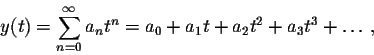in particular y(0)=a0, and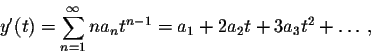in particular y'(0)=a1. This means, we can think of the first two coefficients a0 and a1 as the initial conditions of the differential equation.

How can we evaluate the next coefficient a2? Let us read our recurrence relations for the case n=0: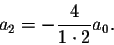Reading off the recurrence relation for n=1 yields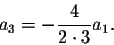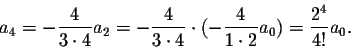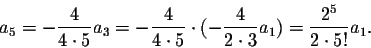What do we know about the solutions to our differential equation at this point? They look like this: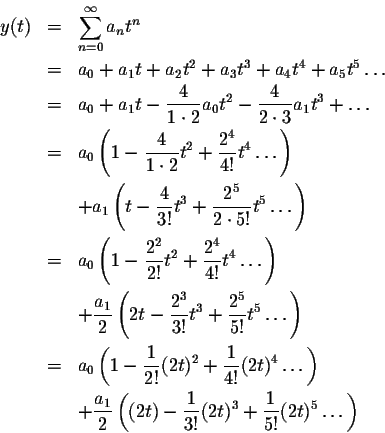Of course the power series inside the parentheses are the familiar functions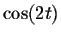and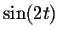: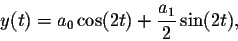so we have found the general solution of the differential equation (with a0 instead of B, and a1/2 instead of A).

The series solutions method is mainly used to find power series solutions of differential equations whose solutions can not be written in terms of familiar functions such as polynomials, exponential or trigonometric functions. This means that in general you will not be able to perform the last few steps of what we just did (less worries!), all we can try to do is to come up with a general expression for the coefficients of the power series solutions.

As another introductory example, let's find the solution to the initial value problem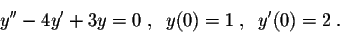The generic form of a power series isWe have to determine the right choice for the coefficients (an).

Start by plugging this "guess" into the differential equation. Recall from the previous section that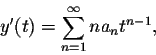andPlugging this information into the differential equation we obtain: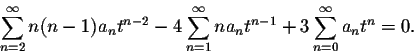Our next goal is to simplify this expression such that only one summation sign "" remains. The obstacle we encounter is that the powers of the three sums are different, tn-2 for the first sum tn-1 for the second sum and tn for the third. We make them the same by shifting the index of the first two sums to obtain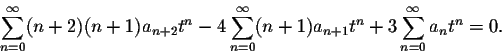Now we can combine the sums as follows: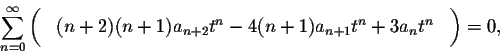and factor out tn: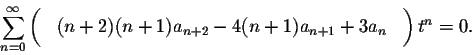Since the power series on the left is identically equal to zero, all of its coefficients are equal to zero: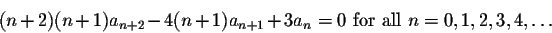Solving these equations for the "highest index" n+2, we can rewrite as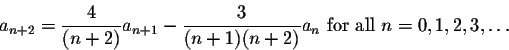These recurrence relations contain all the information about the coefficients we need.

Recall that y(0)=a0 and y'(0)=a1, so our initial conditions imply that a0=1 and a1=2.

Reading off the recurrence relations we can compute the next coefficients: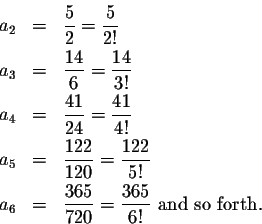Can you see a pattern evolving for the numerators? Going from an to an+1 we multiply by 3 and subtract 1. With mostly patience, experience and some magic, we can see that the nth numerator is of the form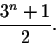This implies that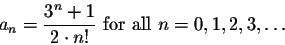This implies that our solution has the power series: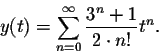We can rewrite this to retrieve the solution in more familiar form: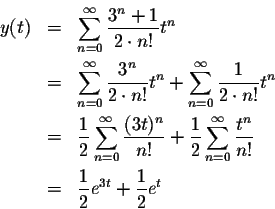Isn't Math fun?[Back] [Next]
[Algebra] [Trigonometry] [Complex Variables]
[Calculus] [Differential Equations] [Matrix Algebra]S.O.S MATHematics home page

Do you need more help? Please post your question on our S.O.S. Mathematics CyberBoard.Helmut Knaust
1998-07-02